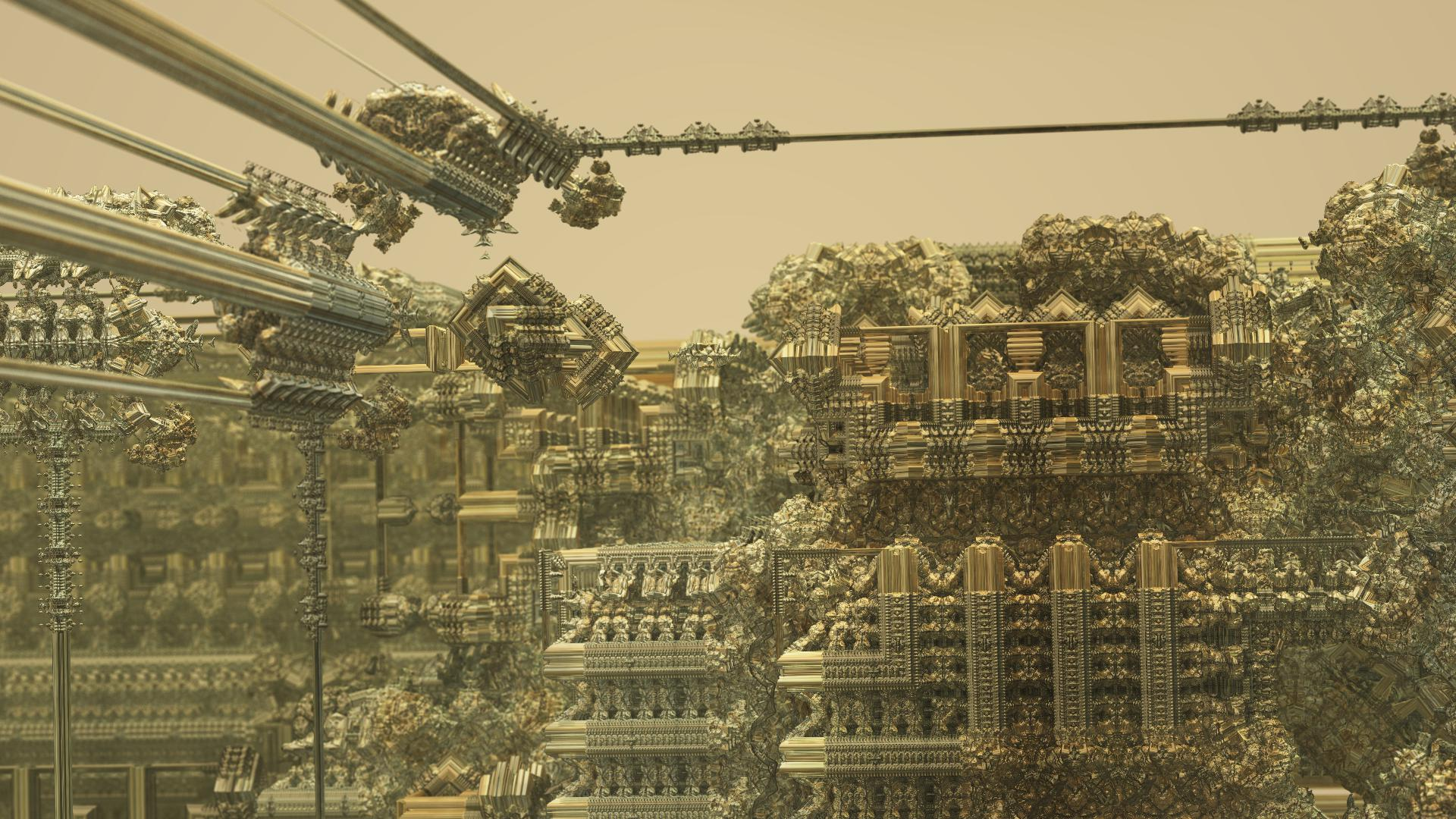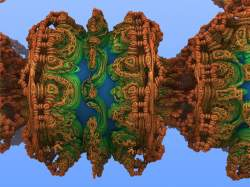News: Support us via Flattr FLATTR Link## The All New FractalForums is now in Public Beta Testing! Visit FractalForums.org and check it out!

preset DefaultPrevious Image | Next Image
Description:  taking a look at the mixpinski.frag at last . great to see so many new frags appearing . thanks to you all : )
Code:
// Created: Wed Nov 16 20:45:18 2016
#define providesInit
#include "MathUtils.frag"
#include "de-Raytracer.frag"

#group MixPinski
//MAIN
uniform float scaleM; slider[0,2,4]
uniform vec4 scaleC; slider[(-5,-5,-5,-5),(1.,1.,.5,.5),(5,5,5,5)]
uniform bool PreOffset; checkbox[false]
uniform vec4 offsetM; slider[(-5,-5,-5,-5),(0,0,0,0),(5,5,5,5)]
uniform float w; slider[-5,0,5]
uniform int MI; slider[0,10,250]
uniform int ColorIterations; slider[0,10,250]

uniform bool ZwLength; checkbox[false]
uniform bool ZwOff; checkbox[false]
uniform int ZwMode; slider[0,03,3]

//Rotations
uniform int PreRotStart; slider[0,1,15]
uniform vec3 PreRotVector; slider[(0,0,0),(1,1,1),(1,1,1)]
uniform float PreRotAngle; slider[0.00,0,180]
uniform int RotStart; slider[0,1,15]
uniform vec3 RotVector; slider[(0,0,0),(1,1,1),(1,1,1)]
uniform float RotAngle; slider[0.00,0,180]

//sphere cut, even works 0_o
uniform int SpStart; slider[0,5,35]
uniform bool SpAbsZwZz; checkbox[false]
//uniform vec2 Cut; slider[(-10,-10),(0,0),(10,10)]

mat3 fracRotation1;
mat3 fracRotation2;
void init() {
fracRotation1 = rotationMatrix3(normalize(PreRotVector), PreRotAngle);
fracRotation2 = rotationMatrix3(normalize(RotVector), RotAngle);
}
// allows varying DE calculation, can remove later

float DE(vec3 p) {
float Dd = 1.0;
vec4 z=vec4(p,w);
float r2=0.;  //  r2 is a better term as we are using radius squared for bailout (dot(z,z) )

int i=0;
for(; i<MI; i++) {
if(PreOffset) z-=offsetM;
if(i>=PreRotStart) z.xyz*=fracRotation1;

if(z.x+z.y<0.0) z.xy = -z.yx;
if(z.x+z.z<0.0) z.xz = -z.zx;
if(z.y+z.z<0.0) z.zy = -z.yz;
if(z.x+z.w<0.0) z.xw = -z.wx;
if(z.y+z.w<0.0) z.yw = -z.wy;
if(z.z+z.w<0.0) z.zw = -z.wz;

z+=offsetM;

z.x= scaleM *z.x-scaleC.x*( scaleM -1.);
z.y= scaleM *z.y-scaleC.y*( scaleM -1.);
z.w= scaleM *z.w-scaleC.w*( scaleM -1.);
z.z-=0.5*scaleC.z*( scaleM -1.)/ scaleM ;
z.z=-abs(-z.z);
if(i>SpStart) {
z.z=min(z.z,sp);
if(SpAbsZwZz)z.w=abs(z.z);
}
z.z+=0.5*scaleC.z*( scaleM -1.)/ scaleM ;
//if(i>SpStart) z.z=min(z.z,sp);
z.z= scaleM *z.z;
//if(i>SpStart) z.z=min(z.z,sp);

if(ZwLength) z.w=length(z.w);
if(ZwMode==1) z.w=cos(z.w);
if(ZwMode==2) z.w-=sin(z.w);
if(ZwMode==3) z.w-=sin(z.w)+cos(z.w);
//if(i>dot(sin(z.x),sin(z.y)))  z.w=length(z.w);

Dd *= scaleM; // for DE calc

if(!ZwOff) r2=z.x*z.x+z.y*z.y+z.z*z.z + z.w * z.w; // bailout criteia, and I added in the z.w part to see if it  necessary
else  r2=z.x*z.x+z.y*z.y+z.z*z.z;
if(i>=RotStart) z.xyz*=fracRotation2;
if (i<ColorIterations) orbitTrap = min(orbitTrap, abs(vec4(z.x,z.y,z.z,z.w)));
}

float r = sqrt(r2);

//return (r - offsetD) / abs(Dd); // offsetD has a default of 0.0 which is the std case. The offsetD results are similar or maybe the same as adjusting Detail Level( Quality)
float Iter = i; // this DE works also in openCL so it might be something to with "i" in Fragmentarium
return r*pow(scaleM,-Iter);
//return r/abs(Dd);
}

#preset Default
FOV = 0.1399457
Eye = 0.4834185,0.814533,0.7137993
Target = -0.6488703,1.176193,-9.068379
Up = 0.0051842,0.9649544,-0.1511864
EquiRectangular = false
AutoFocus = false
FocalPlane = 0.9407666
Aperture = 0.0016563
Gamma = 1.417296
ToneMapping = 5
Exposure = 0.6592951
Brightness = 1.01595
Contrast = 1.427586
Saturation = 0.9778086
GaussianWeight = 1
AntiAliasScale = 0.4929577
DepthToAlpha = false
ShowDepth = false
DepthMagnitude = 0.9381663
Detail = -3.7
DetailAO = -0.0290456
FudgeFactor = 0.1642208
MaxDistance = 1000
MaxRaySteps = 4223
Dither = 0.4818865
NormalBackStep = 0 NotLocked
AO = 0,0,0,0.9503843
Specular = 0.0208768
SpecularExp = 3.056148
SpecularMax = 2.419929
SpotLight = 1,0.9294118,0.6313725,1
SpotLightDir = 0.3559322,0.8135593
CamLight = 0.9372549,1,0.9490196,1.443652
CamLightMin = 1
Glow = 0.2392157,0.1803922,0.4,0.5903022
GlowMax = 282
Fog = 0.7972603
Reflection = 0.5034965 NotLocked
DebugSun = false NotLocked
BaseColor = 0.9686275,0.7058824,0.03529412
OrbitStrength = 1
X = 0.254902,0.04705882,0.01176471,0.9310345
Y = 0.1647059,0.4862745,0.07843137,-0.4317241
Z = 1,0.6980392,0.8588235,0.2248276
R = 0.4,0.7,1,1
BackgroundColor = 0.8745098,0.8117647,0.4941176
CycleColors = true
Cycles = 2.069622
EnableFloor = false NotLocked
FloorNormal = 0,0,1
FloorHeight = 0
FloorColor = 1,1,1
scaleM = 1.988935
scaleC = 0.959806,0.9182259,0.8627859,0.5
PreOffset = true
offsetM = -0.2748782,-0.5532359,-0.4906054,0.0452331
w = 0.0818554
MI = 27
ColorIterations = 14
ZwLength = true
ZwOff = false
ZwMode = 0
PreRotStart = 6
PreRotVector = 1,0.6329562,0.2828854
PreRotAngle = 7.681365
RotStart = 4
RotVector = 0.7734266,1,0.1636364
RotAngle = 127.7215
SpStart = 25
SpAbsZwZz = true
#endpreset

Stats:
Total Favorities: 0 View Who Favorited
Filesize: 393.96kB
Height: 1080 Width: 1920
Discussion Topic: View Topic
Keywords: timemit fragmentarium mixpinski2
Posted by: Tim EmitDecember 15, 2016, 08:20:19 PM

Rating:by 1 members.

 MengerP8 defaultRating:Filesize: 273.69kBDate: May 15, 2010, 01:02:14 AMComments (2)By: Jesse
 Comments (1)3dickulus Global Moderator Fractal SeniorPosts: 1558December 16, 2016, 03:14:33 AMI'm going to have to play with this one a bit now, maybe turn it inside out or slice it for a look inside